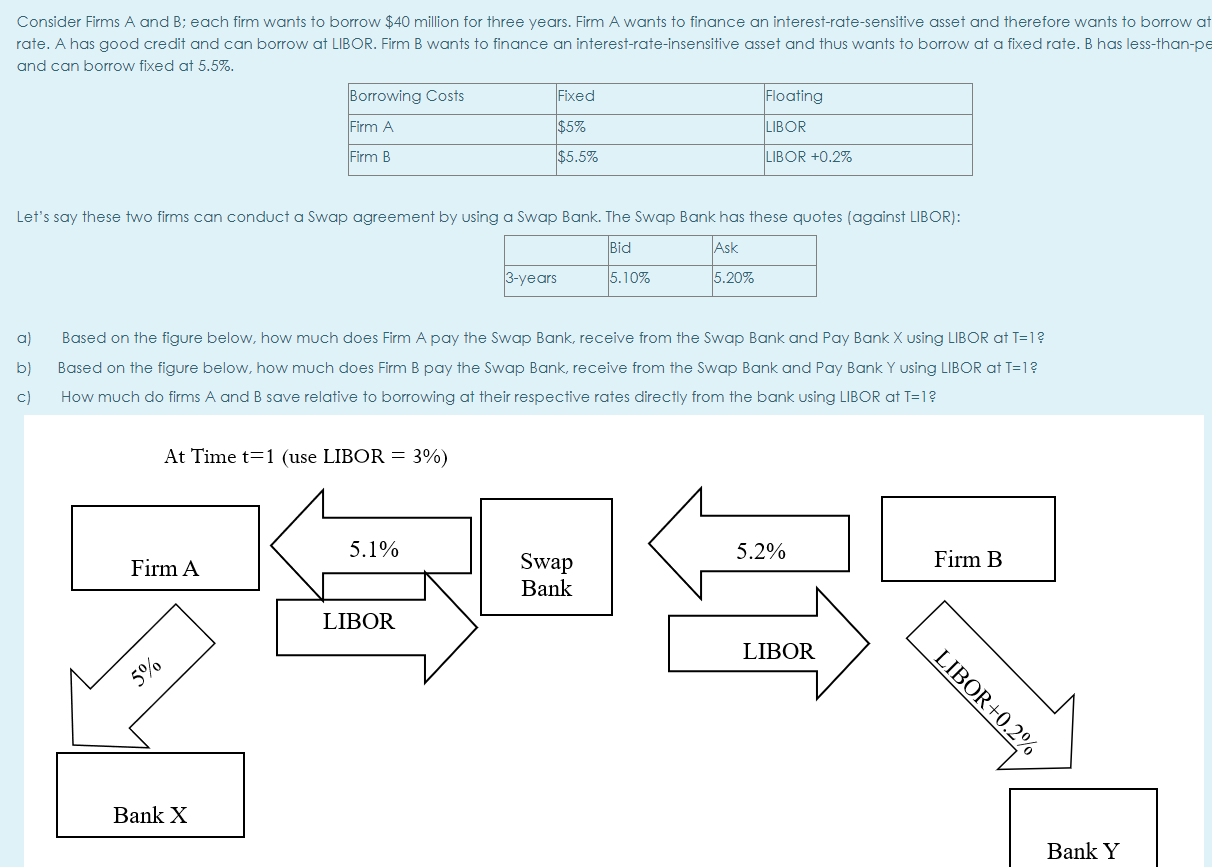# Consider Firms A and B; each firm wants to borrow \$40 million for three yearsConsider Firms A and B; each firm wants to borrow \$40 million for three years. Firm A wants to finance an interest-rate-sensitive asset and therefore wants to borrow at rate. A has good credit and can borrow at LIBOR. Firm B wants to finance an interest-rate-insensitive asset and thus wants to borrow at a fixed rate. B has less-than-pe and can borrow fixed at 5.5%.

Borrowing Costs     Fixed Floating

Firm A                   \$5%                      LIBOR

Firm B                  \$5.5%                LIBOR +0.2%

Let’s say these two firms can conduct a Swap agreement by using a Swap Bank. The Swap Bank has these quotes (against LIBOR):

Bid          Ask

3years    5.10%     5.20%

a) Based on the figure below, how much does Firm A pay the Swap Bank, receive from the Swap Bank and Pay Bank X using LIBOR at T=1 ?

b) Based on the figure below, how much does Firm B pay the Swap Bank, receive from the Swap Bank and Pay Bank Y using LIBOR at T=1 ?

c) How much do firms A and B save relative to borrowing at their respective rates directly from the bank using LIBOR at T=1?

# Click here